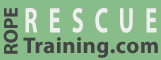# Unit Conversion

It is important to know how to convert between kilograms, kilonewtons, and pounds.

 kilograms (kg or kgf) kilonewtons (kN) newtons (N) pounds (lb or lbf) Equals (mass): Equals (force):

You can use this nifty calculator (which adheres to the mass and force terminology), or you can use the following approximations.

• A pound is a little less than one-half a kilogram (i.e., ~0.45 kg).
• A kilogram is a little more than two pounds (i.e., ~2.2 lb).
• A kilonewton is approximately 100 kilograms-force (i.e., ~225 lbf).

Most climbing and rescue gear is rated in kilonewtons, so it's essential that you can either think in kilonewtons or quickly convert between kilonewtons and your native units. A memory aid for pound-handicapped rescuers is to think of a friend who weighs approximately 225 pounds and nickname them "Kilonewton." Then when you see a piece of equipment rated at, for example, 26 kN, you'll quickly know that gear could hold 26 of your friends.

Working with PowerPoint?
Let vRigger help.